# NCERT Solutions for Class 8 Maths Chapter 2 Exercise 2.3

NCERT Solutions for Class 8 Maths Chapter 2 Exercise 2.3 – Linear Equation in one variable, has been designed by the NCERT to test the knowledge of the student on the topic – Solving Equations having the Variable on both Sides

### NCERT Solutions for Class 8 Maths Chapter 2 Exercise 2.3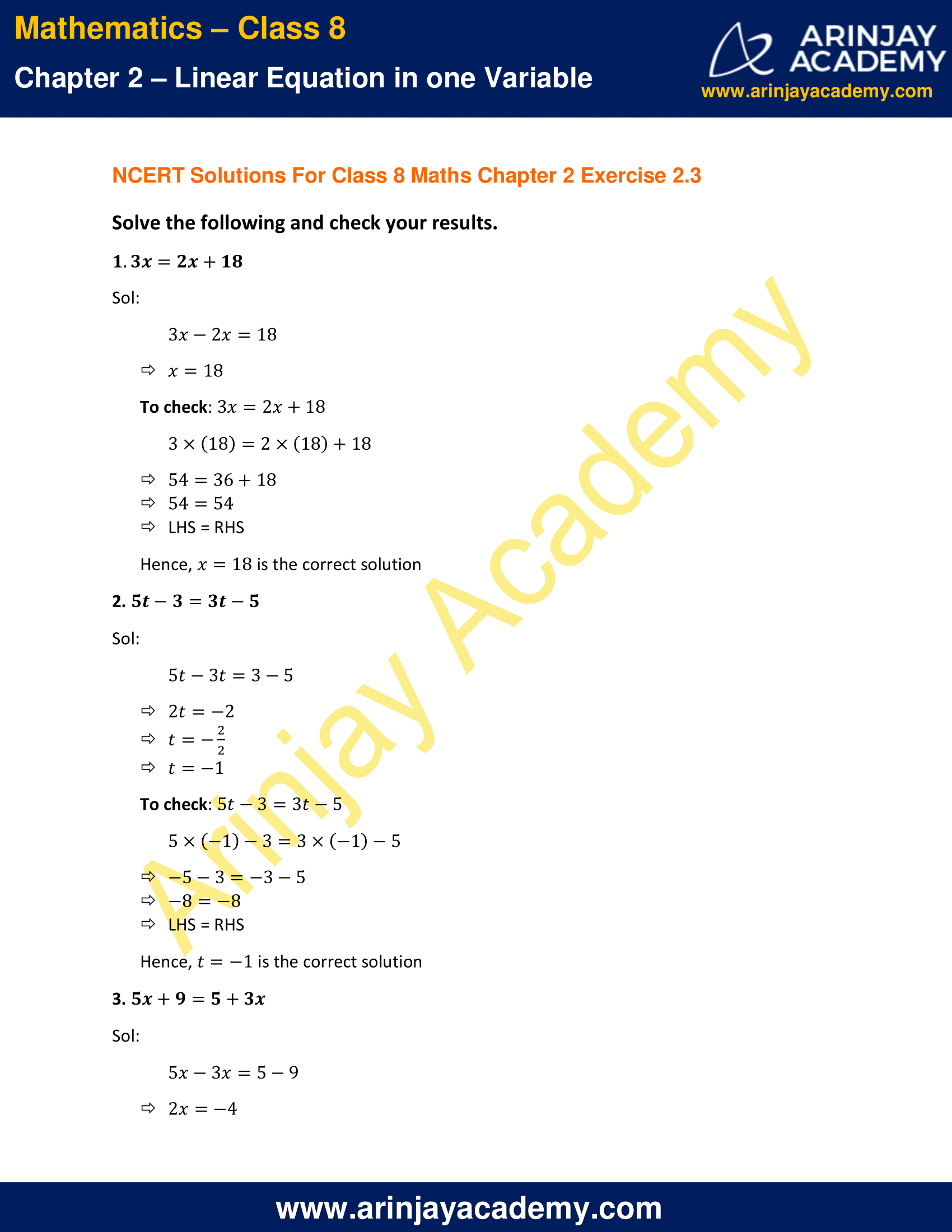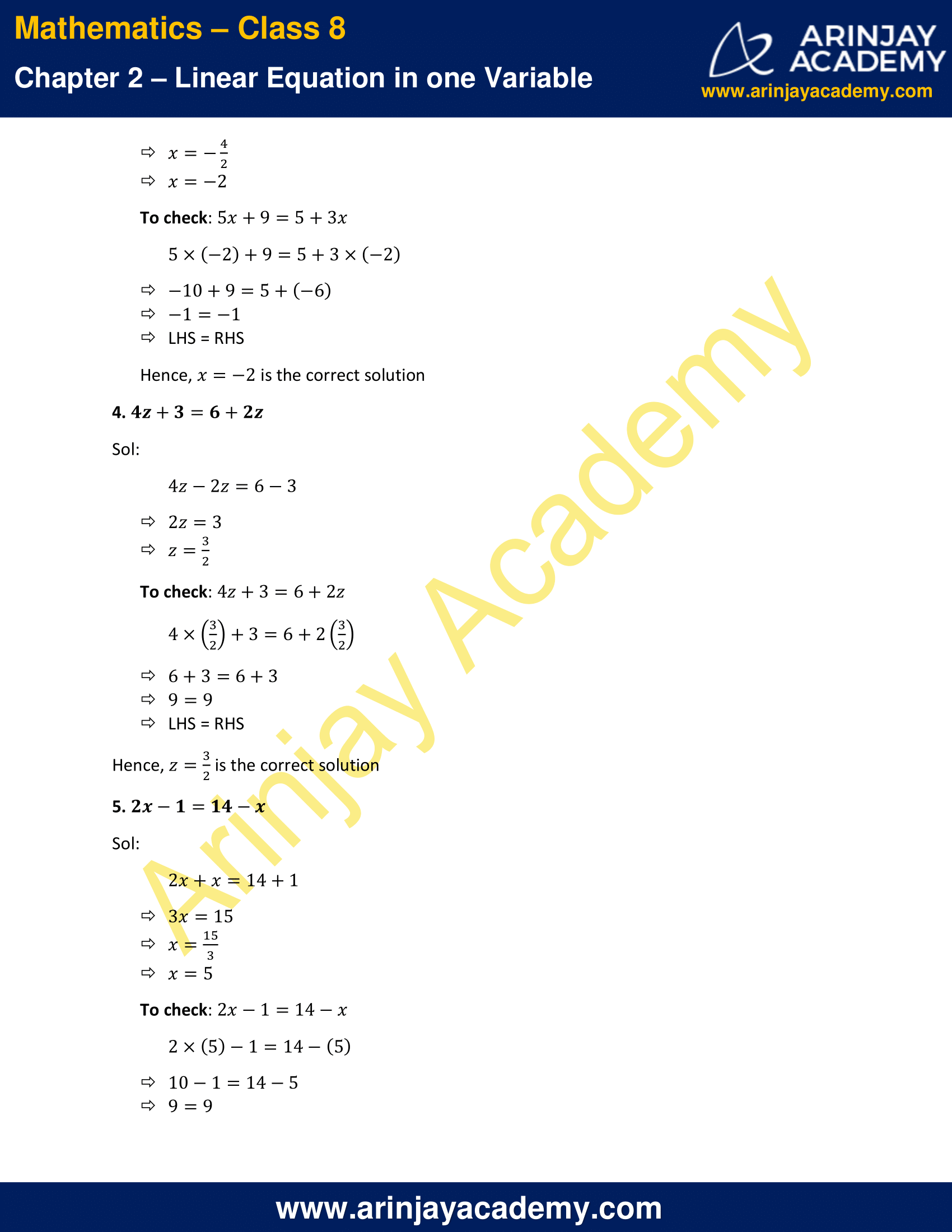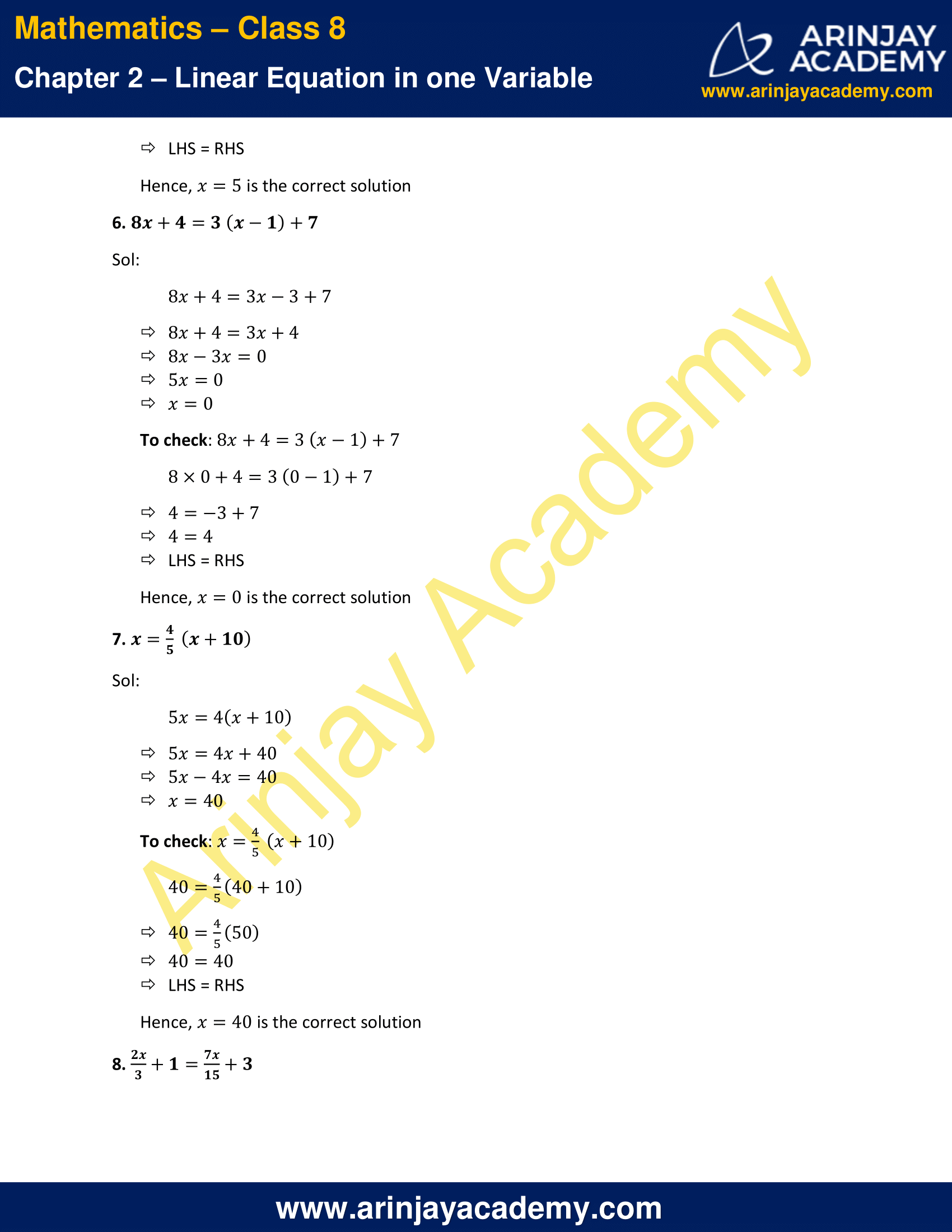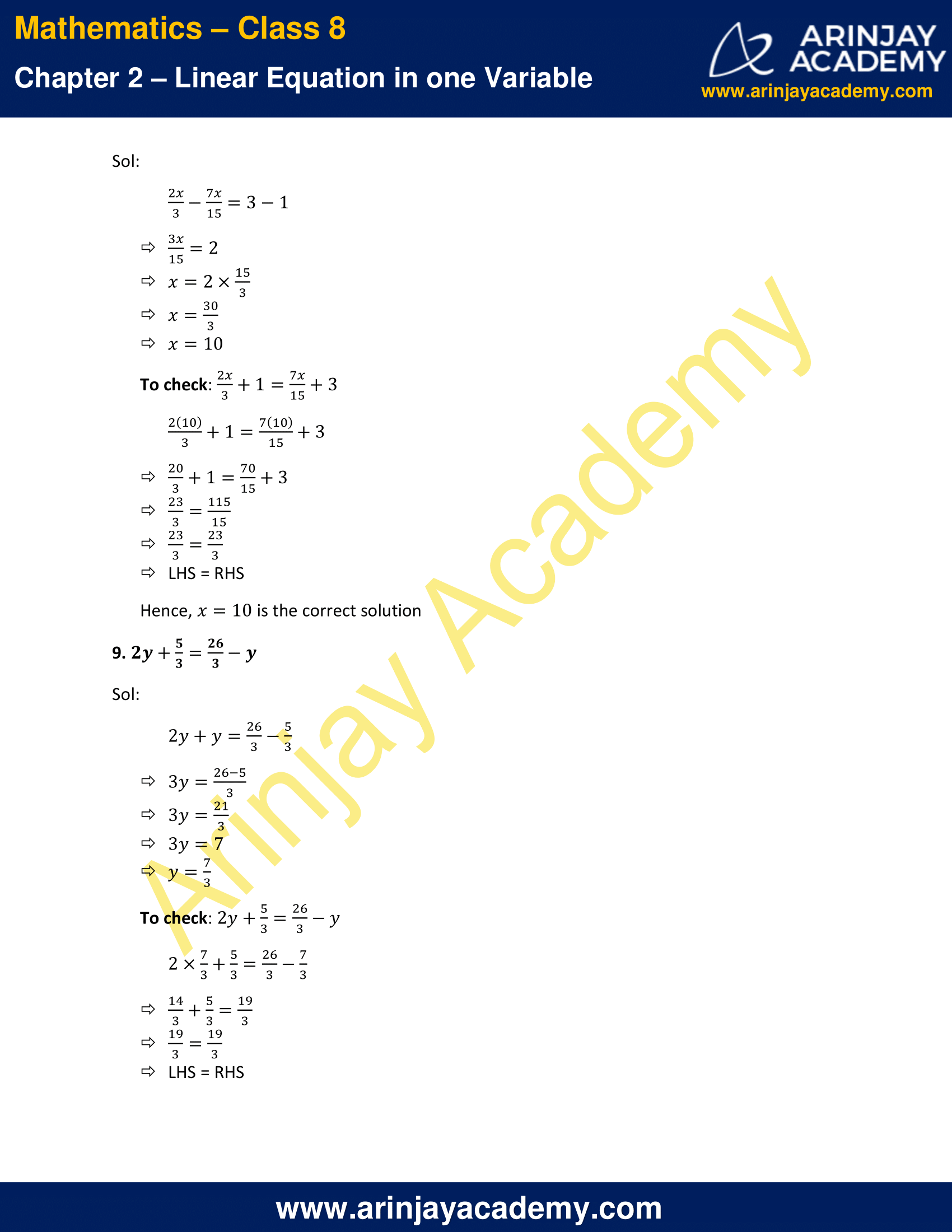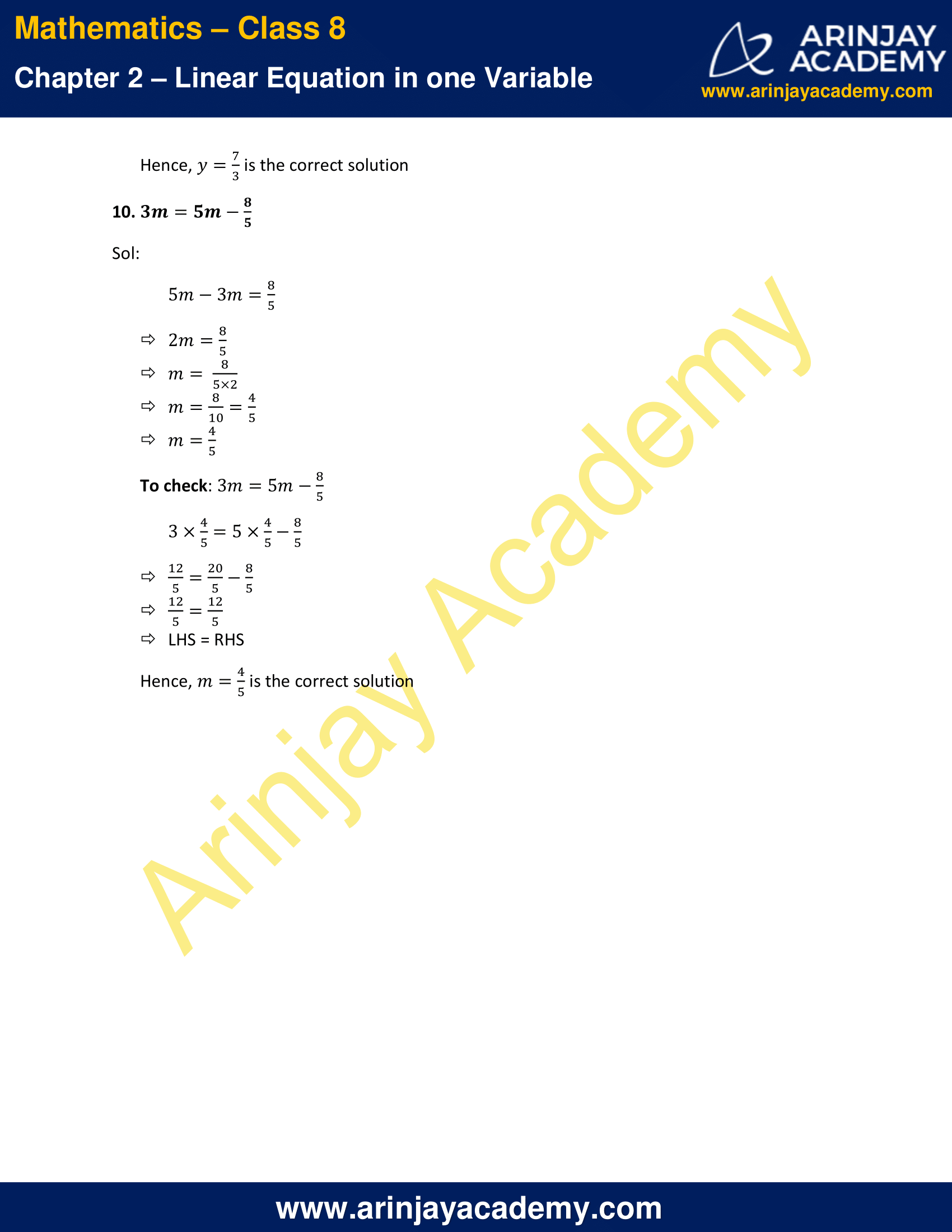NCERT Solutions for Class 8 Maths Chapter 2 Exercise 2.3

Solve the following and check your results.

1. 3x=2x+18

Solution:
3x-2x=18
x=18
To check: 3x=2x+18
3×(18)=2×(18)+18
54=36+18
54=54
LHS = RHS
Hence, x=18 is the correct solution

2. 5t-3=3t-5

Solution:
5t-3t=3-5
2t=-2
t=-(2/2)
t=-1
To check: 5t-3=3t-5
5×(-1)-3=3×(-1)-5
-5-3=-3-5
-8=-8
LHS = RHS
Hence, t=-1 is the correct solution

3. 5x+9=5+3x

Solution:
5x-3x=5-9
2x=-4
x=-(4/2)
x=-2
To check: 5x+9=5+3x
5×(-2)+9=5+3×(-2)
-10+9=5+(-6)
-1=-1
LHS = RHS
Hence, x=-2 is the correct solution

4. 4z+3=6+2z

Solution:
4z – 2z = 6 – 3
2z = 3
z = (3/2)
To check: 4z+3=6+2z
4 × (3/2) + 3 = 6 + 2x(3/2)
6 + 3 = 6 + 3
9 = 9
LHS = RHS
Hence, z = (3/2) is the correct solution

5. 2x-1=14-x

Solution:
2x+x=14+1
3x=15
x=(15/3)
x=5
To check: 2x-1=14-x
2×(5)-1=14-(5)
10-1=14-5
9=9
LHS = RHS
Hence, x=5 is the correct solution

6. 8x+4=3 (x-1)+7

Solution:
8x+4=3x-3+7
8x+4=3x+4
8x-3x=0
5x=0
x=0
To check: 8x+4=3 (x-1)+7
8×0+4=3 (0-1)+7
4=-3+7
4=4
LHS = RHS
Hence, x=0 is the correct solution

7. x=(4/5)(x+10)

Solution:
5x=4(x+10)
5x=4x+40
5x-4x=40
x=40
To check: x=(4/5)(x+10)
40=(4/5)(40+10)
40=(4/5) (50)
40=40
LHS = RHS
Hence, x=40 is the correct solution

8. (2x/3)+1 = (7x/15)+3

Solution:
(2x/3) – (7x/15) = 3-1
(3x/15) = 2
x=(2 × 15)/3
x=(30/3)
x=10
To check: (2x/3)+1=(7x/15)+3
(2 × 10)/3+1=(7 × 10)/15+3
(20/3)+1=(70/15)+3
(23/3)=(115)/15
(23/3) = (23)/3
LHS = RHS
Hence, x=10 is the correct solution

9. 2y + (5/3) = (26/3) – y

Solution:
2y + y = (26/3) – (5/3)
3y = (26-5)/3
3y = (21/3)
3y=7
y=(7/3)
To check: 2y+(5/3)=(26/3)-y
2×(7/3)+(5/3)=(26/3)-(7/3)
(14/3)+(5/3)=(19/3)
(19/3) = (19/3)
LHS = RHS
Hence, y=(7/3) is the correct solution

10. 3m=5m-(8/5)

Solution:
5m-3m=(8/5)
2m=(8/5)
m= (8/5×2)
m = 8/10 = 4/5
m = 4/5
To check: 3m = 5m – (8/5)
3×(4/5)=5×(4/5)-(8/5)
(12/5)=(20/5)-(8/5)
(12/5)=(12/5)
LHS = RHS
Hence, m=(4/5) is the correct solution

The next Exercise for NCERT Solutions for Class 8 Maths Chapter 2 Exercise 2.4 – Linear Equation in one variable can be accessed by clicking here